Pruning

You can choose to prune by the following pattern, which uses the by-metric to choose a node to prune back to a leaf at each iteration until the per-leaf change in the until-metric is operator value times the per-leaf change in the until-metric of replacing the full tree with a single leaf:

PRUNE by-metric / until-metric operator value;


For example, the following statement prunes by average square error until the number of leaves falls below (or is equal to) 3:

PRUNE ASE / N <= 3;


The inequality is necessary because PROC HPSPLIT prunes by removing entire nodes and replacing them with leaves.

The by-metric is used to choose the node to prune back to a leaf. The smallest global increase (or largest decrease) in the specified metric is the criterion used to choose the node to prune. After the pruner chooses the leaf, it uses the until-metric to determine whether to terminate pruning.

For example, consider the following statement.

PRUNE GINI / ENTROPY >= 1.0;


This statement chooses the node with the lowest global change (smallest increase or largest decrease) in the Gini statistic when the node is made into a leaf, and it terminates when removing the node causes a change in global entropy per leaf that is greater than or equal to the per-leaf change in entropy that is caused by replacing the original tree by a single leaf.

To be more precise, if the original tree had an entropy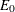and
leaves and trimming away the whole tree to a stump had an entropy of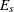(and, because it is a stump, just one leaf), the per-leaf change in entropy is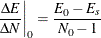If the node, n, that is chosen by the pruner has an entropy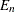and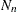leaves and has an entropy of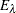if it were replaced by leaf, then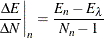The preceding statement would cause the pruner to terminate when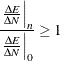For all until-metrics except N, the default operator is >= and the default value is 1.0. For the N until-metric, the default operator is <= and the default value is 5.